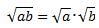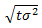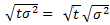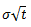# Volatility in Statistics and Markets: Simple Definition, Calculation

Share on

Volatility is a measure of change over time. In statistics, it’s used in a few areas, such as differential equations in the OU process. In stock markets, it quantifies a stock’s lack of stability or the tendency of its prices to move up and down. Usually, the higher the volatility, the riskier the stock.

Mathematically, it’s the standard deviation calculated over a time period; a measure of how much the numbers are spread out around the mean. For stock markets, it is typically given in percentage points.

## Calculating Volatility of Stocks

Volatility is almost always performed on a computer. However, it is possible to calculate volatility by hand, if you know some basic stats.

Standard deviations (σ) are measures of how spread out data is. Therefore, high standard deviations indicate high volatility and low standard deviations equal lower volatility.

The closing price for a stock or index is taken over a certain number of trading days:

• Daily, σdaily, of given stocks, calculate the standard deviation of the daily percentage change for the stocks over a given time period.
• Weekly, σweekly: Calculate the standard deviation of the weekly percentage changes over a time period.
• Annualized, σannual: Since you don’t usually have data from a multi-year period to analyze, you will typically calculate the annualized volatility from either daily or weekly.

As the volatility is calculated from the standard deviation, which is the square root of the variance, we need to multiply by the square root of the time ratio (see note 1 below for why we use the square root of time) in order to switch daily or weekly volatility to annual.

• To change σdaily, to σannualized, multiply the standard deviation of the percentages, calculated for days, by the square root of 252 (based on 252 trading days in a typical year).
• To calculate σannual from the weekly numbers, multiply σweekly, by the square root of 52, as there are 52 weeks in a year.

Suppose you found the daily volatility, σdaily, of a particular stock is 1.2 %. Multiply this by the square root of 252, and you get σannual = 19.05% .

When not specified, a reference to ‘volatility’ is considered to mean σannual.

## Volatility: Lognormal Method in Finance

In financial circles, they like to take the natural log of the numerical change to convert it to a number that is approximately the same as percents. Then, the standard deviation is calculated from that result. In steps, that’s:

1. Find the daily closing price.
2. Calculate the daily logarithmic returns.
3. Find the standard deviation for the returns in step 2, over a specified time period (e.g. the last 7 days). This gives you the daily historical volatility.

## Limitations

While σannual is an important statistic in investment, it does have inherent limitations and weaknesses. Since it is based on the standard deviation, it gives us no information on whether fluctuations tend to be positive or negative, and what the net price change might be.

It is also not a constant; every stock has some periods of high volatility and other periods with comparatively low. There may be more or less variation, but it is never a constant, although for some economic applications (namely the Black Scholes equation) it is assumed to be.

## Why use the square root of time?.

The answer to this question isn’t a simple one, but it has it’s roots in random walks (a.k.a. Brownian motion). What follows is a bare bones mathematical proof of why we use the square root of time; If you’ve got some basic algebra skills, you should be able to follow the logic.

Basically, market prices take random walks. For each increment of a price’s random walk, the variance is proportional to the time taken.
For example:

• Let’s say the variance was equal to 3 in one day. As a proportion, that’s 3/1.
• For day 2, time is doubled, so variance is doubled also, given a variance of 3 * 2 = 6.
• For day 3, time is tripled, so variance is 3 * 3 = 9.

Next, we need a couple of math definitions:

1. The standard deviation is the square root of variance.
2. The product of square roots rule states that the square root of a product equals the square root of each factor in the product, multiplied together (Study.com).We can use the above definitions to make a new formula:

1. Replacing a in the formula with variance (σ2) and b with time (t).2. Using the product of square roots rule to separate time and variance.3. Finally, we know that the standard deviation is the square root of variance, so we can replace the √σ2 with σ.## References

Kotz, S.; et al., eds. (2006), Encyclopedia of Statistical Sciences, Wiley.
Ladokhin, Sergiy. Forecasting σ in the Stock Market. Retrieved from http://citeseerx.ist.psu.edu/viewdoc/download;jsessionid=633DF5B24D9D52C3200A582C6636BBF9?doi=10.1.1.501.2747&rep=rep1&type=pdf on June 17, 2018< Macroption. Why Is Volatility Proportional to the Square Root of Time? Retrieved June 25, 2018 from: http://www.macroption.com/why-is-volatility-proportional-to-square-root-of-time/ How to Calculate σannual: Putting market vol.into annual terms. The Motley Fool. Retrieved from https://www.fool.com/knowledge-center/how-to-calculate-annualized-volatility.aspx on June 17, 2018.
Study.com. Product of Square Roots Rule: Definition & Example. Retrieved June 25, 2018 from: https://study.com/academy/lesson/product-of-square-roots-rule-definition-example.html

CITE THIS AS:
Stephanie Glen. "Volatility in Statistics and Markets: Simple Definition, Calculation" From StatisticsHowTo.com: Elementary Statistics for the rest of us! https://www.statisticshowto.com/volatility/
---------------------------------------------------------------------------Need help with a homework or test question? With Chegg Study, you can get step-by-step solutions to your questions from an expert in the field. Your first 30 minutes with a Chegg tutor is free!